# matrix

## The Alon-Tarsi basis conjecture ★★

Author(s): Alon; Linial; Meshulam

Conjecture   Ifare invertible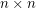matrices with entries in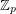for a prime, then there is asubmatrixof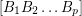so thatis an AT-base.

## The permanent conjecture ★★

Author(s): Kahn

Conjecture   Ifis an invertiblematrix, then there is ansubmatrixofso that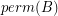is nonzero.

Keywords: invertible; matrix; permanent

## The additive basis conjecture ★★★

Author(s): Jaeger; Linial; Payan; Tarsi

Conjecture   For every prime, there is a constant(possibly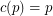) so that the union (as multisets) of anybases of the vector spacecontains an additive basis.